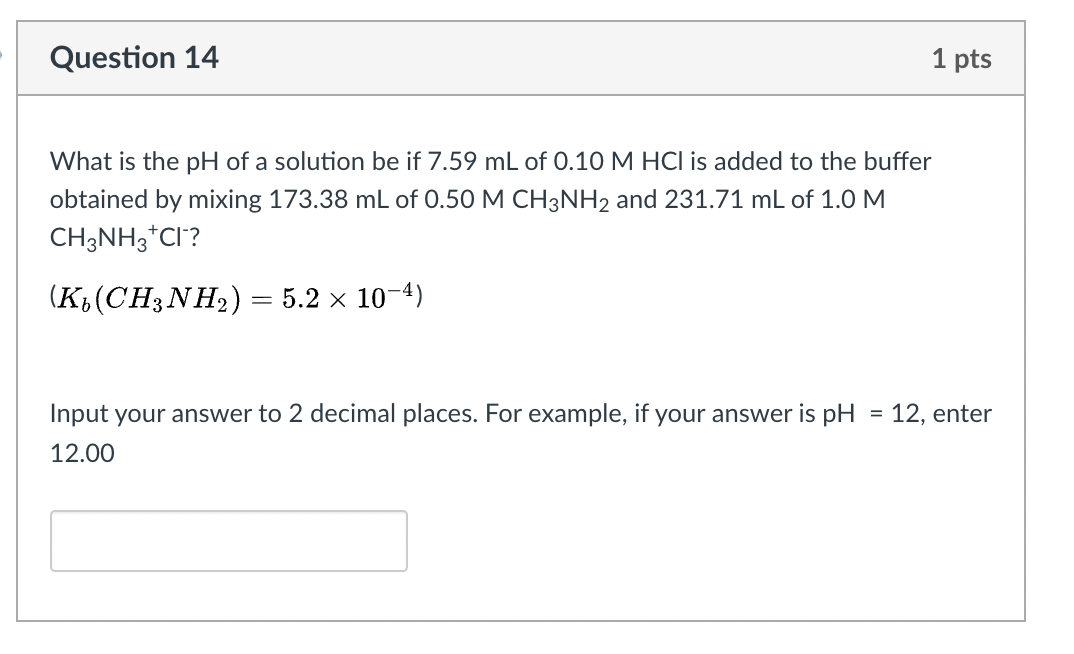# Question Solved1 AnswerQuestion 14 1 pts What is the pH of a solution be if 7.59 mL of 0.10 M HCl is added to the buffer obtained by mixing 173.38 mL of 0.50 M CH3NH2 and 231.71 mL of 1.0 M CH3NH3CI? (K(CH3NH2) = = 5.2 x 10-4) = 12, enter Input your answer to 2 decimal places. For example, if your answer is pH 12.00Transcribed Image Text: Question 14 1 pts What is the pH of a solution be if 7.59 mL of 0.10 M HCl is added to the buffer obtained by mixing 173.38 mL of 0.50 M CH3NH2 and 231.71 mL of 1.0 M CH3NH3CI? (K(CH3NH2) = = 5.2 x 10-4) = 12, enter Input your answer to 2 decimal places. For example, if your answer is pH 12.00
More
Transcribed Image Text: Question 14 1 pts What is the pH of a solution be if 7.59 mL of 0.10 M HCl is added to the buffer obtained by mixing 173.38 mL of 0.50 M CH3NH2 and 231.71 mL of 1.0 M CH3NH3CI? (K(CH3NH2) = = 5.2 x 10-4) = 12, enter Input your answer to 2 decimal places. For example, if your answer is pH 12.00